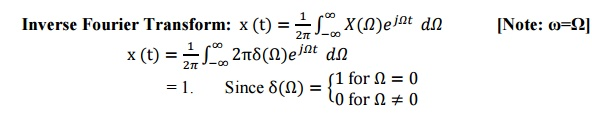Home | | Signals and Systems | Important Short Questions and Answers: Analysis of Continuous Time Signals

# Important Short Questions and Answers: Analysis of Continuous Time Signals

Signals and Systems - Analysis of Continuous Time Signals - Important Short Questions and Answers: Analysis of Continuous Time Signals

1.  What are the DirichletŌĆÖs conditions of Fourier series?

(i).The function x(t) should be single valued within the interval T0

(ii). The function x(t) should have atmost a finite number of discontinuities in the interval T0

(iii). The function x(t) should have finite number of maxima and minima in the interval T0

(iv). The function should have absolutely integrable.

2.  State any two properties of continuous time Fourier transform.

Convolution (Time) Property:

It states that,

x (t)*y(t) Ōåö(FT)X (jŌä”)Y(jŌä”)

Modulation Property (or) Frequency Shifting:

It states that,3.  Find the laplace transform of the signal4. What are the difference between Fourier series and Fourier transform?

Fourier Series

1. Fourier series is calculated for periodic signals.

2. Expands the signals in time domain.

3. Three types of Fourier series such as trigonometric, Polar and Complex Exponential

Fourier Transform

1. Fourier Transform is calculated for non-periodic as well as periodic signals.

2. Represents the signal in frequency Domain

3. Fourier transform has no such types.

5.  State initial and final value theorem of Laplace transform.6. Define the Fourier transform pair for continuous time signal. (Or) Give synthesis  and analysis equations of CT Fourier Transform.7.  Find inverse Fourier transform of X(Žē)=2ŽĆ╬┤ (Žē).8.  State the time scaling property of Laplace Transform.9.  What is the Fourier Transform of a DC signal of amplitude 1?10. Define region of convergence of the Laplace Transform.11. State the relationship between fourier transform and laplace transform.ŌĆó The laplace transform is same as fourier transform when s =jŽē

12.      State any two properties of ROC of laplace transform X(s) of a signal x(t).

Properties of ROC:

┬Ę        No poles lie in ROC.

┬Ę        ROC of the causal signal is right hand sided. It is of the form Re(s)>a.

┬Ę        ROC of the non causal signal is left hand sided. It is of the form Re(s) < a.

┬Ę        The system is stable if its ROC includes jŽē axis of s-plane.

13. Determine fourier series coefficients for signal cos14. Give analysis and synthesis equations of fourier transform.15. Obtain the fourier transform of X(f) = e-atu(t) , a > 016. What is the condition to be satisfied for the existence of fourier transform for CT periodic signals?

The function x(t) should be absolutely integrable for     the existence of    fourier transform.Study Material, Lecturing Notes, Assignment, Reference, Wiki description explanation, brief detail
Signals and Systems : Analysis of Continuous Time Signals : Important Short Questions and Answers: Analysis of Continuous Time Signals |